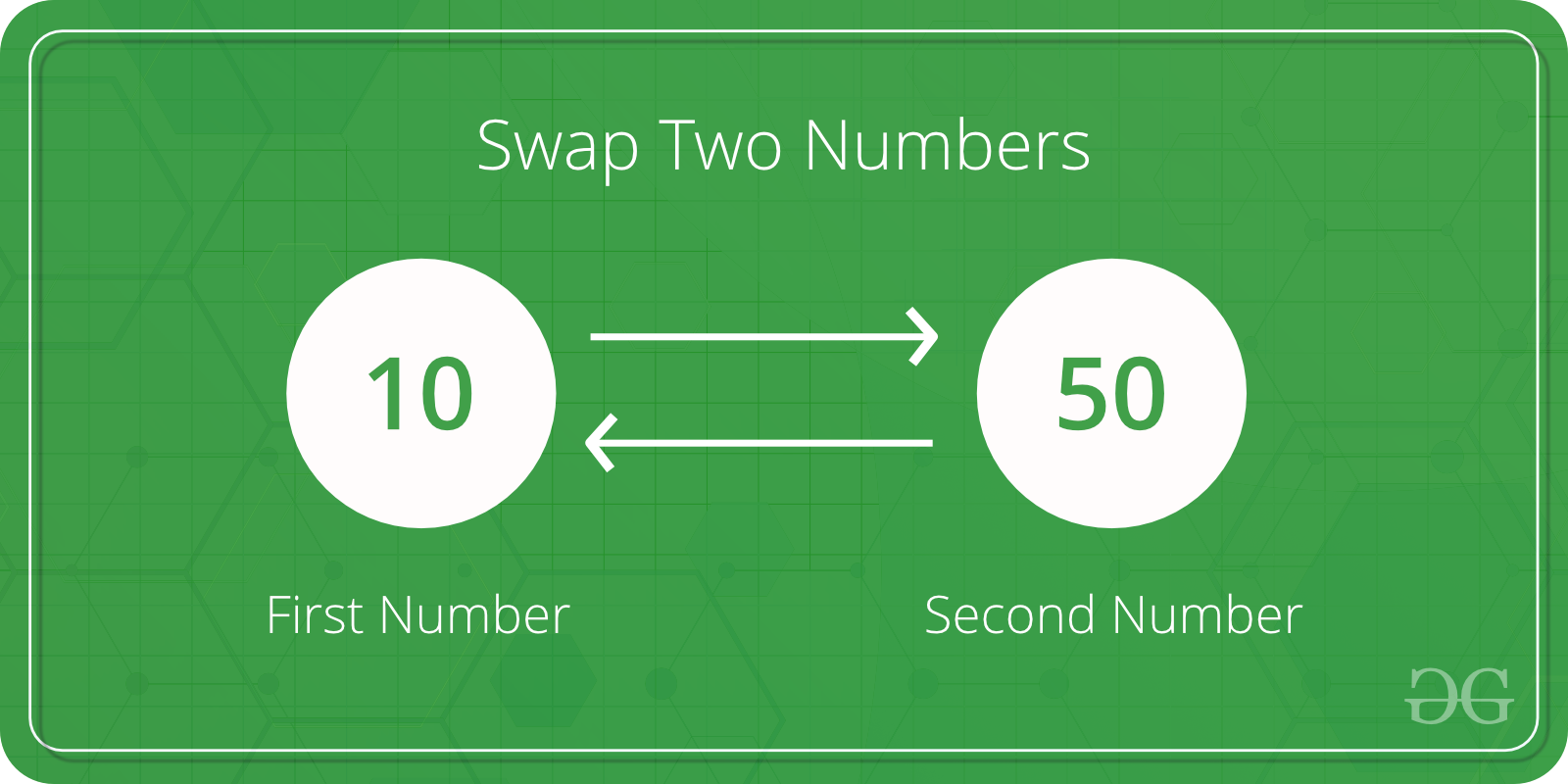# C Program to Swap two Numbers

Swapping two numbers means exchanging their values. In this article, we will learn how to swap two numbers in the C programming language.## Swap Two Numbers Using a Temporary Variable

Below is the C program to swap two numbers using a temporary variable.

## C

 `// C program to swap two variables` `#include `   `// Driver code` `int` `main()` `{` `    ``int` `x, y;` `    ``printf``(``"Enter Value of x "``);` `    ``scanf``(``"%d"``, &x);` `    ``printf``(``"\nEnter Value of y "``);` `    ``scanf``(``"%d"``, &y);`   `    ``// Using a temporary variable to swap the values` `    ``// Store the value of x in a temporary variable` `    ``int` `temp = x;` `    ``// Assign the value of y to x` `    ``x = y;` `    ``// Assign the value stored in the temporary variable to` `    ``// y` `    ``y = temp;`   `    ``printf``(``"\nAfter Swapping: x = %d, y = %d"``, x, y);` `    ``return` `0;` `}`

Output

```Enter Value of x
Enter Value of y
After Swapping: x = 0, y = 0```

### Complexity Analysis

• Time Complexity: O(1)
• Auxiliary Space: O(1)

### Explanation

We swap two numbers by storing the value of one number in a temporary variable, assigning the value of the second number to the first number, and then assigning the value stored in the temporary variable to the second number.

## Swap Two Numbers Without Using a Temporary Variable

Below is the C/C++ program to swap two numbers without using a temporary variable.

## C

 `// C program to swap two numbers` `// without using a third variable` `#include `   `// Driver code` `int` `main()` `{` `    ``int` `a, b;` `    ``printf``(``"Enter the two numbers : \n"``);` `    ``scanf``(``"%d %d"``, &a, &b);` `    ``printf``(``"Before swapping A is : %d and B is %d \n"``, a,` `           ``b);`   `    ``// Swapping without using a third variable` `    ``// Sum of both numbers is stored in 'a'` `    ``a = a + b;` `    ``// Difference of sum and original 'b' is` `    ``// stored in 'b'` `    ``b = a - b;` `    ``// Difference of sum and new 'b' is stored in` `    ``// 'a'` `    ``a = a - b;`   `    ``printf``(``"After swapping A is : %d and B is : %d \n"``, a,` `           ``b);` `    ``return` `0;` `}`

Output

```Enter the two numbers :
Before swapping A is : 0 and B is 32767
After swapping A is : 32767 and B is : 0 ```

### Complexity Analysis

• Time Complexity: O(1)
• Auxiliary Space: O(1)

### Explanation

The idea is to use arithmetic operators. We will take the sum of the two numbers and store it in one number and store the difference of both numbers in the other number. Finally, we will store the difference of both numbers in the first number. Since the values will be updated after the first two operations we will be able to swap the numbers.

Refer to the complete article on How to swap without using a temporary variable? for more details!

Feeling lost in the world of random DSA topics, wasting time without progress? It's time for a change! Join our DSA course, where we'll guide you on an exciting journey to master DSA efficiently and on schedule.
Ready to dive in? Explore our Free Demo Content and join our DSA course, trusted by over 100,000 geeks!

Previous
Next